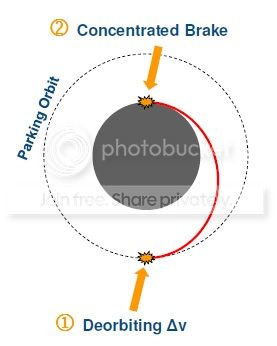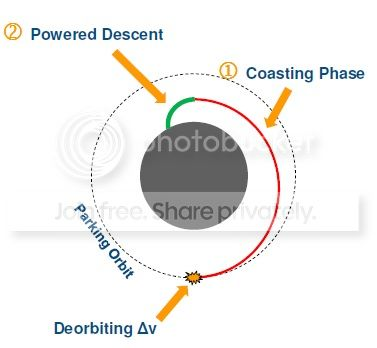# Landing Satellite via Hohmann Transfer

I'm working on a project that is dealing with calculating the orbital motion of satellites around some given body and one thing I was wanting to try and study was the path that a satellite would take to land on the body it was orbiting. I'm wondering if it's possible to use the concept of Hohmann transfers to calculate the trajectory of the satellite as it lands. Do you all think that this would be an acceptable way to approach this problem or is there a more efficient way to do this? Thanks for any help.

• It'd be helpful if you described your scenario in more detail. Does the planet the sat is landing on have an atmosphere? What is the altitude of the sat's starting orbit? – HopDavid May 10 '14 at 13:59

I'm wondering if it's possible to use the concept of Hohmann transfers to calculate the trajectory of the satellite as it lands. Do you all think that this would be an acceptable way to approach this problem or is there a more efficient way to do this?

The answer is "no", and it has nothing to do with efficiency.

For simplicity, let's assume the target body is spherical. A Hohmann transfer to the surface of that body would result in an orbit just above the surface of the body. That orbital rate is inevitably going to differ markedly from the velocity at which a point on the surface of the target body is moving. You don't want to be orbiting the body centimeters above the surface. You want to be landing on the body, and that means making your velocity equal to the body's rotational velocity rather than that orbital velocity.

• Thanks for your reply. What if when I fire my rocket the second time, instead of firing it so that my velocity matches a circular orbit velocity I fire it in such a way that my velocity matches the body's rotational velocity. That should be a lower value than the circular orbit velocity which should allow me to actually land on the body. Is this a possible solution or am I grasping at straws with the whole thing? – John May 8 '14 at 12:12
• You can't treat landing as an impulsive process. If the target body doesn't have an atmosphere, instead think of it as the reverse of a launch. Things get dicier if the target body does have an atmosphere. – David Hammen May 8 '14 at 13:47
• If aerobraking is part of the part of the EDL you'd want a small flight path angle as you hit the atmosphere. To get a low flight path angle you'd want a perigee near earth's surface. – HopDavid May 10 '14 at 13:57

To minimize gravity drag you want to minimize fighting gravity. I basically means that you want to transfer to an orbit just above the surface. Depending on you initial trajectory this might be a Hohmann transfer, or maybe a bi-elliptical transfer.

After this you would want to perform a constant altitude landing. I am not sure if this is the correct technical term, since I have only heard this being by Kerbal Space Program players, when I into this myself. This was also being discussed in this forum thread.

The amount of fuel/change in velocity this would require would depend on your (initial) thrust to weight ratio (TWR) and engine efficiency (since less efficient engine would burn more mass, making it lighter, thus its TWR will increase faster assuming a constant exerted force). For this I also assume that the rate at which the pitch/direction of the thrust vector of the satellite has to changes would not be a problem. So the thrust should be angled in such a way that all vertical "force" add to zero (using a rotating reference frame, thus also include the centripetal force). So the angle of the thrust with the vertical, $\theta$, can be calculated as follows,

$$\frac{F}{m}\sin{\theta}=\frac{\mu}{r^2}-\frac{v^2}{r},$$ where $F$ is the thrust, $m$ the mass, $v$ the orbital velocity (so not necessarily relative to the surface) and $r$ the radius of the trajectory of the satellite relative to the center of the celestial body, and $\mu$ the gravitational parameter of the celestial body. So when solving for $\theta$ yields, $$\theta=\sin^{-1}\left(\frac{m}{F}\left(\frac{\mu}{r^2}-\frac{v^2}{r}\right)\right),$$ however $m$ and $v$ are time dependent, so a differential equation will have to be solved. Namely by using the rate at which fuel is burned, $$\dot{m} = \frac{F}{v_e},$$ where $v_e$ is the effective exhaust velocity. And horizontal component of the thrust, $$\dot{v} = \frac{F}{m}\cos{\theta}.$$ I do not believe this differential equation can be solved analytically, so numerical methods will have to be used.

How I currently formulated these equations would assume that you are landing along the equator of the celestial body, either against or with its rotation, since you would have to use a stopping criteria for $v$ when your relative velocity with respect to the surface is zero. I also assumed that there are no peaks and valleys in the terrain (the celestial body is completely in hydrostatic equilibrium). If there would be then you either would have to end up exactly on top of a peak, or drop down a bit into a valley and perform an additional burn to slow down from that.

Just to add some $\Delta v$ preliminary estimation in the case of a landing performed with a "Hohmann-like" 2-impulses manoeuvre.

Let's assume we start from a 100 km parking orbit and we want to land on the Moon (assumed as perfectly spherical).

$\Delta v_{tot} = \Delta v_{1} + \Delta v_{2}$

$\Delta v_{1} = \sqrt{\dfrac{\mu}{r_{park}}} - \sqrt{2 \mu \biggl( \dfrac{1}{r_{park}} - \dfrac{1}{r_{park}+r_{moon}} \biggr)} = 22.99 \ m/s$

$\Delta v_{2} = \sqrt{2 \mu \biggl( \dfrac{1}{r_{moon}} - \dfrac{1}{r_{park}+r_{moon}} \biggr)} = 1702.40 \ m/s$

$\Delta v_{tot} = 1725.39 \ m/s$The issues related to this manoeuvre are:

• too tight trajectory

• not vertical touchdown (the $\Delta v$ will not be directed in the vertical direction)

A better approach would be:

• impulsive de-orbiting burn on an intermediate transfer orbit

• coasting phase (ballistic)

• powered descent phase